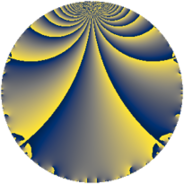# Properties

 Label 6552.2.a.gLevel $6552$ Weight $2$ Character orbit 6552.a Self dual yes Analytic conductor $52.318$ Analytic rank $0$ Dimension $1$ CM no Inner twists $1$

# Related objects

Show commands: Magma / PariGP / SageMath

## Newspace parameters

comment: Compute space of new eigenforms

[N,k,chi] = [6552,2,Mod(1,6552)]

mf = mfinit([N,k,chi],0)

lf = mfeigenbasis(mf)

from sage.modular.dirichlet import DirichletCharacter

H = DirichletGroup(6552, base_ring=CyclotomicField(2))

chi = DirichletCharacter(H, H._module([0, 0, 0, 0, 0]))

N = Newforms(chi, 2, names="a")

//Please install CHIMP (https://github.com/edgarcosta/CHIMP) if you want to run this code

chi := DirichletCharacter("6552.1");

S:= CuspForms(chi, 2);

N := Newforms(S);

 Level: $$N$$ $$=$$ $$6552 = 2^{3} \cdot 3^{2} \cdot 7 \cdot 13$$ Weight: $$k$$ $$=$$ $$2$$ Character orbit: $$[\chi]$$ $$=$$ 6552.a (trivial)

## Newform invariants

comment: select newform

sage: f = N # Warning: the index may be different

gp: f = lf \\ Warning: the index may be different

 Self dual: yes Analytic conductor: $$52.3179834043$$ Analytic rank: $$0$$ Dimension: $$1$$ Coefficient field: $$\mathbb{Q}$$ Coefficient ring: $$\mathbb{Z}$$ Coefficient ring index: $$1$$ Twist minimal: yes Fricke sign: $$-1$$ Sato-Tate group: $\mathrm{SU}(2)$

## $q$-expansion

comment: q-expansion

sage: f.q_expansion() # note that sage often uses an isomorphic number field

gp: mfcoefs(f, 20)

 $$f(q)$$ $$=$$ $$q - 2 q^{5} + q^{7}+O(q^{10})$$ q - 2 * q^5 + q^7 $$q - 2 q^{5} + q^{7} + 2 q^{11} - q^{13} + 6 q^{17} + 4 q^{19} + 2 q^{23} - q^{25} - 4 q^{31} - 2 q^{35} + 2 q^{37} - 2 q^{41} - 4 q^{43} + q^{49} + 12 q^{53} - 4 q^{55} + 6 q^{61} + 2 q^{65} - 12 q^{67} + 2 q^{71} - 2 q^{73} + 2 q^{77} + 4 q^{83} - 12 q^{85} - 2 q^{89} - q^{91} - 8 q^{95} - 10 q^{97}+O(q^{100})$$ q - 2 * q^5 + q^7 + 2 * q^11 - q^13 + 6 * q^17 + 4 * q^19 + 2 * q^23 - q^25 - 4 * q^31 - 2 * q^35 + 2 * q^37 - 2 * q^41 - 4 * q^43 + q^49 + 12 * q^53 - 4 * q^55 + 6 * q^61 + 2 * q^65 - 12 * q^67 + 2 * q^71 - 2 * q^73 + 2 * q^77 + 4 * q^83 - 12 * q^85 - 2 * q^89 - q^91 - 8 * q^95 - 10 * q^97

## Embeddings

For each embedding $$\iota_m$$ of the coefficient field, the values $$\iota_m(a_n)$$ are shown below.

For more information on an embedded modular form you can click on its label.

comment: embeddings in the coefficient field

gp: mfembed(f)

Label $$\iota_m(\nu)$$ $$a_{2}$$ $$a_{3}$$ $$a_{4}$$ $$a_{5}$$ $$a_{6}$$ $$a_{7}$$ $$a_{8}$$ $$a_{9}$$ $$a_{10}$$
1.1
 0
0 0 0 −2.00000 0 1.00000 0 0 0
 $$n$$: e.g. 2-40 or 990-1000 Significant digits: Format: Complex embeddings Normalized embeddings Satake parameters Satake angles

## Atkin-Lehner signs

$$p$$ Sign
$$2$$ $$1$$
$$3$$ $$1$$
$$7$$ $$-1$$
$$13$$ $$1$$

## Inner twists

This newform does not admit any (nontrivial) inner twists.

## Twists

By twisting character orbit
Char Parity Ord Mult Type Twist Min Dim
1.a even 1 1 trivial 6552.2.a.g 1
3.b odd 2 1 6552.2.a.t yes 1

By twisted newform orbit
Twist Min Dim Char Parity Ord Mult Type
6552.2.a.g 1 1.a even 1 1 trivial
6552.2.a.t yes 1 3.b odd 2 1

## Hecke kernels

This newform subspace can be constructed as the intersection of the kernels of the following linear operators acting on $$S_{2}^{\mathrm{new}}(\Gamma_0(6552))$$:

 $$T_{5} + 2$$ T5 + 2 $$T_{11} - 2$$ T11 - 2 $$T_{17} - 6$$ T17 - 6

## Hecke characteristic polynomials

$p$ $F_p(T)$
$2$ $$T$$
$3$ $$T$$
$5$ $$T + 2$$
$7$ $$T - 1$$
$11$ $$T - 2$$
$13$ $$T + 1$$
$17$ $$T - 6$$
$19$ $$T - 4$$
$23$ $$T - 2$$
$29$ $$T$$
$31$ $$T + 4$$
$37$ $$T - 2$$
$41$ $$T + 2$$
$43$ $$T + 4$$
$47$ $$T$$
$53$ $$T - 12$$
$59$ $$T$$
$61$ $$T - 6$$
$67$ $$T + 12$$
$71$ $$T - 2$$
$73$ $$T + 2$$
$79$ $$T$$
$83$ $$T - 4$$
$89$ $$T + 2$$
$97$ $$T + 10$$